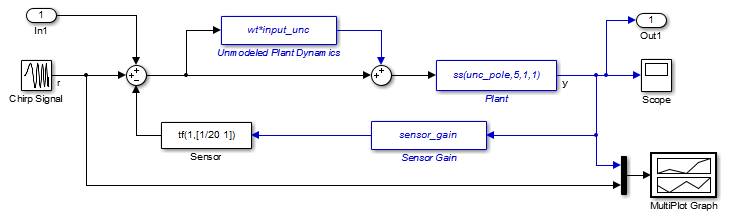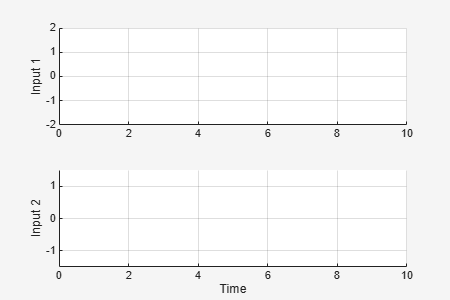# ufind

Find uncertain variables in Simulink model

## Syntax

``uvars = ufind(mdl)``
``[uvars,pathinfo] = ufind(mdl)``
``uvars = ufind(usys1,...,usysN)``

## Description

example

````uvars = ufind(mdl)` finds Uncertain State Space blocks in the Simulink® model `mdl`. The function returns a structure containing all uncertain variables associated with the Uncertain State Space blocks. These uncertain variables are the `ureal`, `umargin`, or `ultidyn` elements in the `uss` model specified in the Uncertain system variable parameters of the blocks.`ufind` finds Uncertain State Space blocks inside masked subsystems, library links, and model references. However, the function does not find blocks inside accelerated model references. ```

example

````[uvars,pathinfo] = ufind(mdl)` returns paths to each Uncertain State Space block in `mdl` and the corresponding uncertain variables referenced by the block. You can use this information to verify that all Uncertain State Space blocks have been identified.```
````uvars = ufind(usys1,...,usysN)` collects uncertain variables referenced by the uncertain dynamic system models `usys``1,…,usysN`, which can be `uss` or `ufrd` models. You can use this syntax as a faster alternative to querying the Simulink model, when you know the uncertain models referenced by its Uncertain State Space blocks.```

## Examples

collapse all

Find all Uncertain State Space blocks and uncertain variables in a Simulink® model. First, open the model.

```mdl = "usim_model"; open_system(mdl)```The model contains three Uncertain State Space blocks named `Unmodeled Plant Dynamics`, `Plant`, and `Sensor Gain`. These blocks are predefined to depend on three uncertain variables named `input_unc`, `unc_pole` and `sensor_gain`. Use `ufind` to find all these uncertain variables in the model and gather them into a data structure.

`[uvars,pathinfo] = ufind(mdl);`Examine the structure `uvars`. Each uncertain variable is a `ureal` or `ultidyn` uncertain element and is listed by name in `uvars`.

`uvars`
```uvars = struct with fields: input_unc: [1x1 ultidyn] sensor_gain: [1x1 ureal] unc_pole: [1x1 ureal] ```

You can use `uvars` for analysis of the responses of the uncertain system. For instance, you can use it as an argument to `usample` to simulate the model at random values of all the uncertain variables, as shown in Vary Uncertain Values Across Multiple Uncertain Blocks.

If you need a list of the block paths that contain uncertain variables, use the `pathinfo` output argument. `pathinfo` is a two-column cell array. The first column lists the paths to each Uncertain State Space block in the model. The second column lists the uncertain elements associated with the corresponding block.

`pathinfo`
```pathinfo = 3x2 cell {'usim_model/Plant' } {'unc_pole' } {'usim_model/Sensor Gain' } {'sensor_gain'} {'usim_model/Unmodeled Plant Dynamics'} {'input_unc' } ```

## Input Arguments

collapse all

Simulink model name, specified as a string or a character vector.

If the same uncertain variable name has different definitions in `mdl`, the function returns an error. For example, if your model contains two Uncertain State Space blocks, one referencing a variable `'unc_par'` with value `ultidyn('unc_par',[1 1])` and another referencing `'unc_par'` with value `ureal('unc_par',5)`, an error occurs.

Example: `"usim_model"`, `'usim_model'`

Uncertain dynamic system model, specified as a `uss` model object or a `ufrd` model object.

## Output Arguments

collapse all

Uncertain variables in the input model, returned as a structure whose fields are the names of the uncertain variables in the model and whose values are the uncertain elements. These uncertain variables are:

• The uncertain elements in any `uss` or `ufrd` models referenced in the Uncertain State Space blocks in the Simulink model specified by `mdl`.

• The uncertain elements in the `uss` or `ufrd` models specified by `usys``1,…,usysN`.

Paths to blocks referencing uncertain variables, returned as a two-column cell array. The first column lists the paths to each Uncertain State Space block in the model, specified as character vectors. The second column lists the uncertain variables associated with the corresponding Uncertain State Space block, also specified as character vectors.

## Version History

Introduced in R2009b